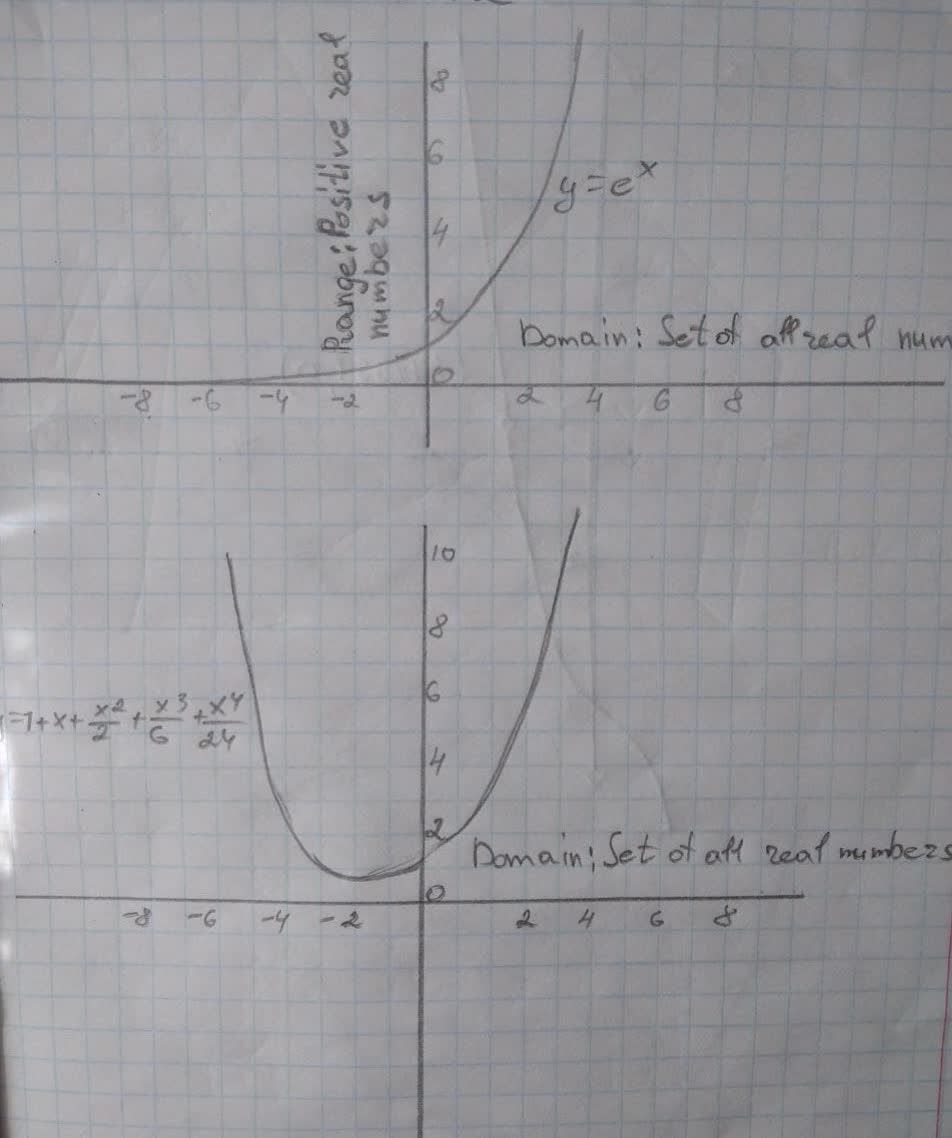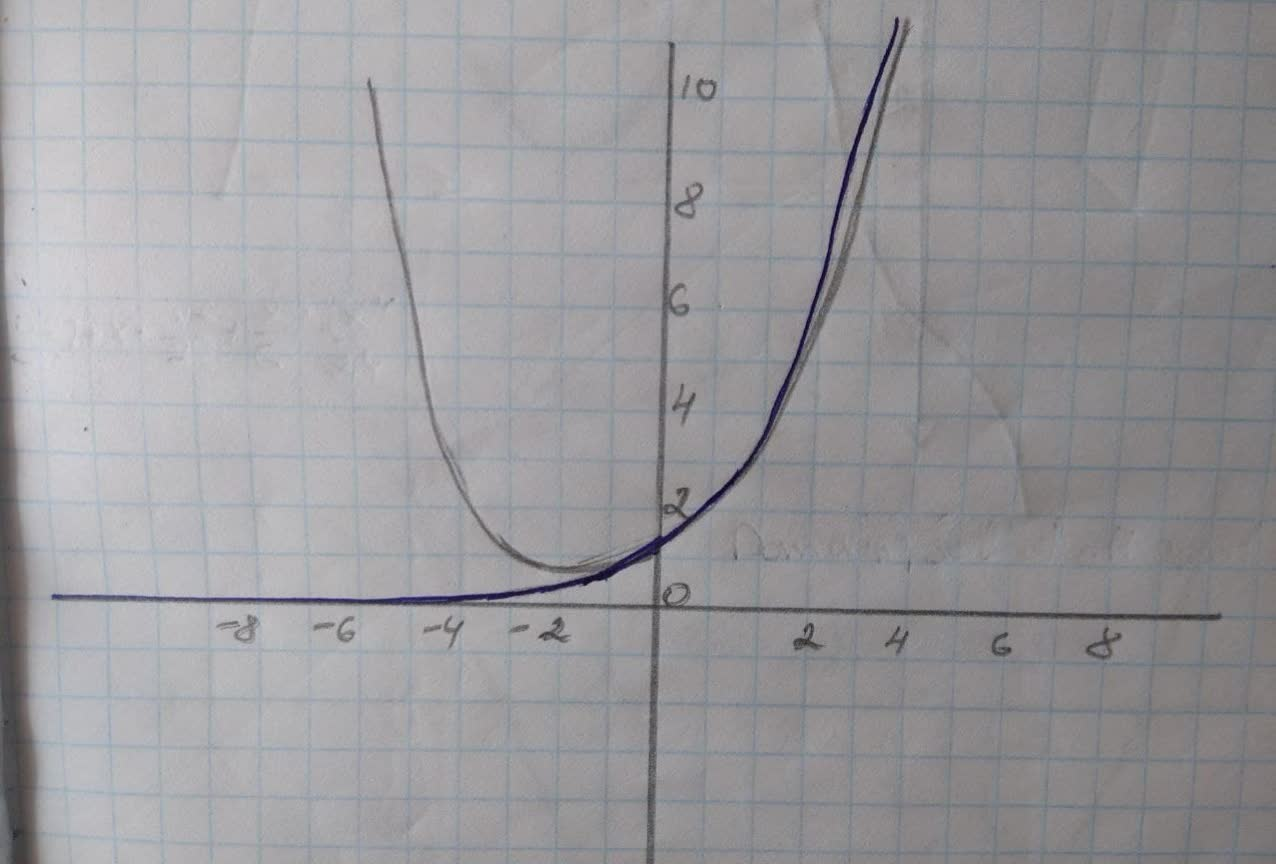Graph y= e^{x}\ and\ y=1+x+x^{2}/2 + x^{3}/6 +x^{4}/24 in theMichelle Isakson 2021-12-03 Answered
Graph $$\displaystyle{y}={e}^{{{x}}}\ {\quad\text{and}\quad}\ {y}={1}+{x}+\frac{{x}^{{{2}}}}{{2}}+\frac{{x}^{{{3}}}}{{6}}+\frac{{x}^{{{4}}}}{{24}}$$ in the same viewing rectangle.

• Questions are typically answered in as fast as 30 minutes

Solve your problem for the price of one coffee

• Math expert for every subject
• Pay only if we can solve itLinda Tincher
Two functions are given that, first is $$\displaystyle{y}={e}^{{{x}}}$$ and anotheris $$\displaystyle{y}={1}+{x}+{\frac{{{x}^{{{2}}}}}{{{2}}}}+{\frac{{{x}^{{{3}}}}}{{{6}}}}+{\frac{{{x}^{{{4}}}}}{{{24}}}}$$.
The graph of exponential functional $$\displaystyle{y}={e}^{{{x}}}$$ is:The graph of polynomial functional $$\displaystyle{y}={1}+{x}+{\frac{{{x}^{{{2}}}}}{{{2}}}}+{\frac{{{x}^{{{3}}}}}{{{6}}}}+{\frac{{{x}^{{{4}}}}}{{{24}}}}$$..
The graphs of both functions in the same viewing rectangle are: